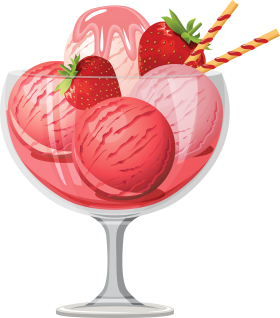# Consumer Equilibrium In Case of a Single Commodity

## Consumer Equilibrium

The state of balance obtained by an end-user of products that refers to the number of goods and services they can buy given their existing level of income and the prevailing level of cost prices. Consumer equilibrium permits a customer to get the most satisfaction possible from their income.

Related Link: Theory Of Consumer Behaviour

(A) Meaning Of Consumer’s Equilibrium Equilibrium means a state of maximum satisfaction.

Consumer’s equilibrium is a situation when he spends his given income on the purchase of one or more commodities in such a way that he gets maximum satisfaction and has no urge to change this level of consumption, given the prices of commodities.

(B) Condition Of Consumer Equilibrium In Case Of Single Commodity The consumer will be in the state of equilibrium when the following condition is fulfilled:

The marginal utility of commodity ‘X’ in terms of rupees is equal to the price of commodity ‘X’ in rupees. [MUx (in Rs.) = Px (Rs.)]

Or

Mux (in utils) = Px (in Rs.) or MU of Commodity ‘X’ (in utils) = Px in Rs)
MUm (in utils) MU of Money (a rupee)(in utils)

(C) Hypothetical Schedule/ Numerical Example Let us take the example of Fruit Ice-cream. Price of an ice-cream Scoop is Rs.30 and MUm i.e. MU of money (Re 1) = 1 util.

 Units ConsumedMU of Ice-cream Scoop (in utils) {a} MU of Money (Re 1) (in utils) {b} MU of Ice-Cream Scoop (in Rs.) = {a}/ {b} Price of Ice-Cream Scoop (Rs.) 1 2 3 4 5 50 40 30 20 10 1 1 1 1 1 50 40 30 20 10 > > = < < 30 30 30 30 30
(D) Explanation And Conclusion In the given example, the level of Consumer’s Equilibrium is 3 units
where,
MU of Ice Cream in rupees = Price of Ice Cream in rupees i.e. Rs.30.

• Before this level, i.e. at 1st and 2nd level, MU > Price, i.e. benefit is more than cost. So, the consumer will increase consumption to attain equilibrium.
• After this level, i.e. at 4th and 5th level, MU < Price, i.e. benefit is less than cost. So, the consumer will cut or decrease consumption to be in the state of equilibrium. Only at the level of 3 units, the condition of consumer’s equilibrium is fulfilled.
• A consumer will consume that much quantity at which MUx = Px to be in the state of equilibrium.
 Important Topic in Commerce:

### Lets Practice

1. If MUx> Px, the consumer
1. Has reached the equilibrium
2. Has started incurring losses
3. Can consume more of X
4. Must stop consuming X

Answer: 3) Can consume more of X

2. A consumer gets maximum satisfaction where the ____________are same.
1. Total utility and marginal utility
2. Price of commodity and marginal utility
3. Price of commodity and total utility
4. (a) and (b) both

Answer: 2) Price of commodity and marginal utility

3.Equilibrium means state of maximum _____________ ?

1. prabhashvari

very understandable lesson

2. IQRA

Well explained

3. Evidence

This is self-explanatory. Well done!!

4. abhi mishra

appreciated